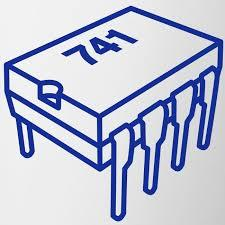# Operational Amplifier And 555 Timer A1 And A3

10 Questions | Attempts: 106
ShareSettings• 1.
Pick all incorrect statements. Analog signals are A. Continuous. B. Easy to process compared to digital signals. C. More immune to Noise compared to digital signals. D. Easily reproducible when compared to digital signals.
• A.

A, B and D

• B.

B, C and D

• C.

B and C

• D.

B and D

• 2.
Find the gain of the inverting amplifier shown below. Consider R1 = 1kΩ and RF = 10kΩ Assume op-amp is ideal.
• A.

-10

• B.

-10k

• C.

-100

• D.

-1

• 3.
Open loop gain of an IC 741 is
• A.

2*10^5

• B.

1

• C.

• D.

0

• 4.
In the application of OP-AMP as an amplifier _____________ feedback is used
• A.

Positive

• B.

No

• C.

Negative

• D.

Series

• 5.
In given circuit Vin = 2 V, R1 = 1KΩ and R2 = 10KΩ.What is the output voltage of an amplifier
• A.

11 V

• B.

-22 V

• C.

22 V

• D.

-20 V

• 6.
For first order butterworth high pass filter, calculate lower cut off frequency if R=15KΩ and C = 0.01. Also calculate Avf if Rf = 10K Ω and R1 = 5kΩ.
• A.

1061 Hz,3

• B.

1061Hz,2

• C.

1061kHz,3

• D.

1061KHz,2

• 7.
Output produce by an op-amp is 180 degree phase shifted when input is applied to
• A.

Inverting terminal

• B.

Non Inverting terminal

• C.

Both inverting and non inverting terminals

• D.

Common mode

• 8.
Determine closed loop voltage gain of an non inverting amplifier if feedback resistor is 3 times input resistor
• A.

3

• B.

6

• C.

4

• D.

-3

• 9.
Voltage follower is also called as
• A.

Unity gain buffer

• B.

Non inverting amplifier

• C.

Buffer

• D.

Inverting amplifier

## Related TopicsBack to top
×

Wait!
Here's an interesting quiz for you.